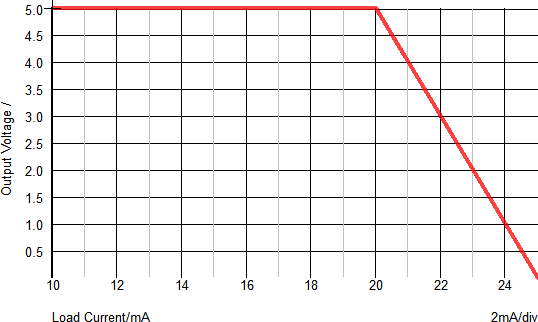Parameterized Linear Regulator

The Parameterized Linear Regulator models a linear regulator with an adjustable maximum output and short circuit current.The model is implemented as a two segment piecewise linear device where the first segment ramps the output voltage to follow the input voltage and the second segment has a slope near 0 to model the output voltage in regulation.

In this topic:

 Model Name: Linear Regulator With Line & Load Regulation and turn-on/-off Simulator: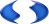This device is compatible with the SIMPLIS simulator Parts Selector Menu Location: Analog Functions > Linear Regulators > With Line & Load Regulation and turn-on/-off Symbol Library: simplis_analog_functions.sxslb Model File: simplis_analog_functions.lb Symbol Name: SIMPLIS_LINEAR_REG Subcircuit Name: SIMPLIS_LINEAR_REG_BB Symbol: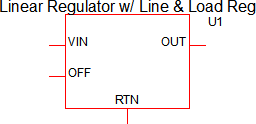Multiple Selections: Multiple devices can be selected and edited simultaneously.

Previous Version Compatibility

This model and symbol was introduced with version 8.2; however, the model and supporting files are identical to the Parameterized Voltage Reference.

For a version of the Parameterized Voltage Reference editable with versions prior to version 7.20, see the parts selector entry:

Obsolete > Voltage References > Voltage Reference With Line & Load Regulation and turn-on/-off (Obsolete)

Editing the Parameterized Linear Regulator

To configure the Linear Regulator, follow these steps:

1. Double click the symbol on the schematic to open the editing dialog.
2. Change the I/O Characteristics and Parameters.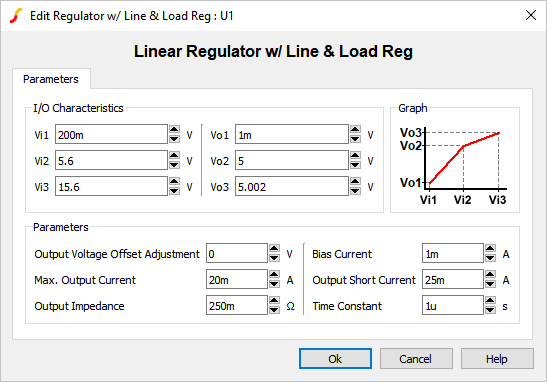Parameter Label Units Description Vi1 V First input voltage point that defines the response characteristic. Vi2 V Second input voltage point that defines the response characteristic. Vi3 V Third input voltage point that defines the response characteristic. Vr1 V First output voltage point that defines the response characteristic. Vr2 V Second output voltage point that defines the response characteristic. Vr3 V Third output voltage point that defines the response characteristic. Output Voltage Offset Adjustment V DC offset for the regulated output voltage. Max. Output Current A Maximum output current. If the output current exceeds this value, the output voltage will start to decrease. Output Impedance Ω Output impedance of the reference. Bias Current A Input bias current. Output Short Current A Current supplied by the reference during an output short circuit event. Time Constant s Internal time constant.

Examples

Below are two examples which demonstrate certain aspects of the linear regulator.

Example 1 - Startup Behavior

The test circuit used to generate the waveform examples in the next section can be downloaded here: simplis_083_linear_reg_ramp_input.sxsch.Example 1 - Waveforms

The waveforms shown below are for a test circuit with a ramped input voltage. A brief description of the behavior is as follows, with the dialog below used for reference:• At t=0μs, the input voltage (Vi) starts at 0V and begins to ramp up to a final voltage of 8V.
• At t=25μs, the input voltage will reach 200mV (Vi1 above). This will correlate to an output voltage of 1mV (Vr1 above).
• At t=700μs, the input voltage will reach 5.6V (Vi2 above). This will correlate to an output voltage of 5V (Vr2 above). At this point, the regulator is at the regulation point.
• At t>700μs, the output voltage will remain ~5V and will rise linearly based on Vi3 and Vr3. In a typical application, the input voltage range from Vi2 to Vi3 is when the output is in regulation.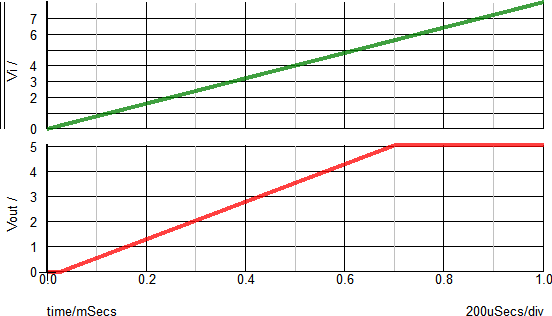The X-Y plot below helps to visualize the relationship between the input voltage (Vi) and the output voltage (Vr). The X-Axis shows the input voltage and the Y-Axis show the regulated output voltage. With no load applied, the inflection points should closely match the Vi and Vo points defined in the dialog.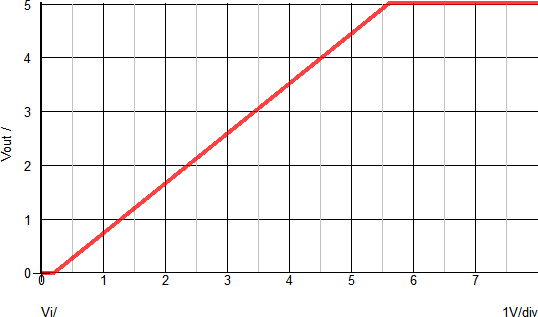Example 2 - Ramped Load

The test circuit used to generate the waveform examples in the next section can be downloaded here: simplis_083_linear_reg_ramp_load.sxsch.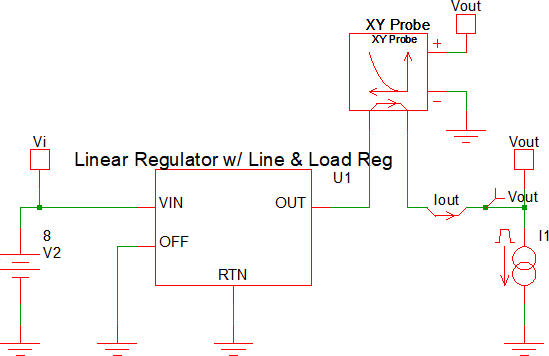Example 2 - Waveforms

The waveforms shown below are for a test circuit with a ramped load current. A brief description of the behavior is as follows, with the dialog below used for reference:• At t=0μs, the load current starts at 10mA.
• At t=250μs, the load current starts to ramp and continues to a final value of 25mA.
• At t=~580μs, the load current reaches the Max. Output Current value of 20mA.
• At t>580μs, the regulated output voltage will begin to decrease as the load current increases.
• At t=750μs, the load current will reach the Output Short Circuit current value of 25mA. At this point, the output voltage will be ~0V.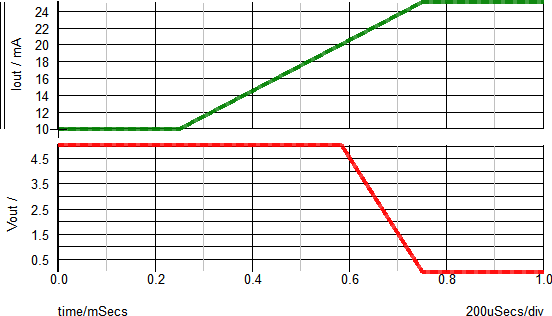The X-Y plot below helps to visualize the relationship between the regulated output voltage and the output current. The X-Axis shows the output voltage and the Y-Axis show the load current. You can see that as the load current increases above the Max. Output Current value (20mA), the output voltage will decrease.